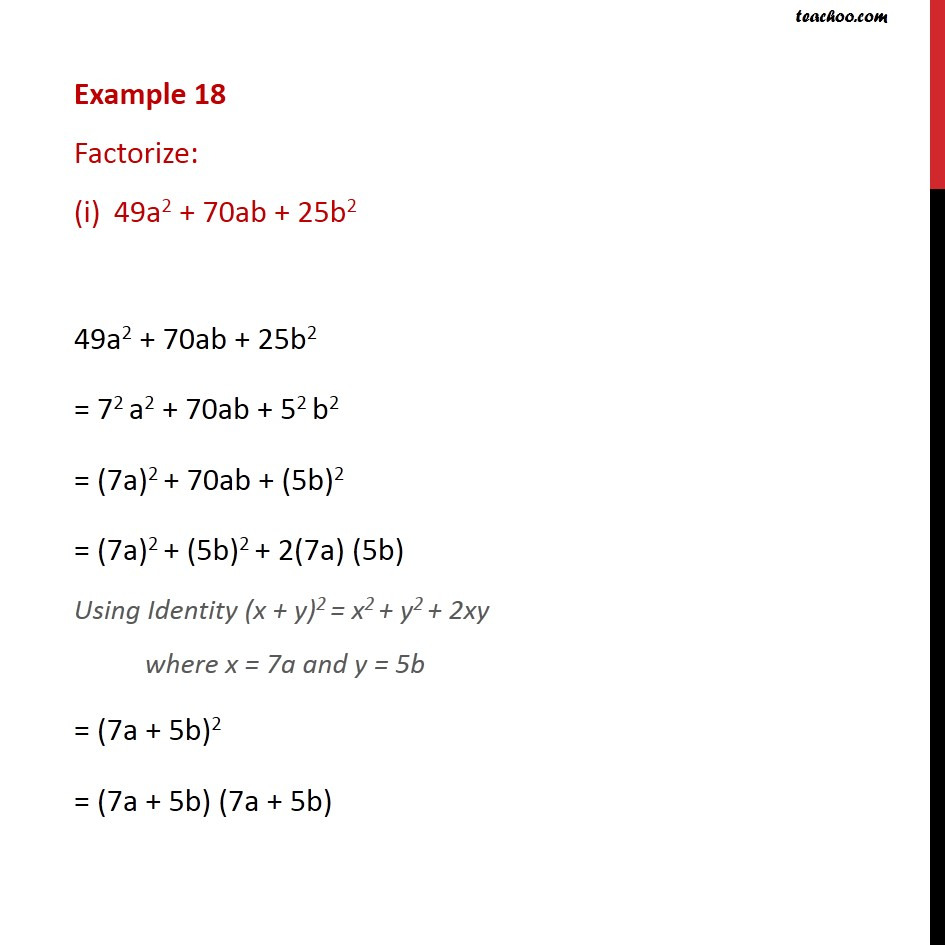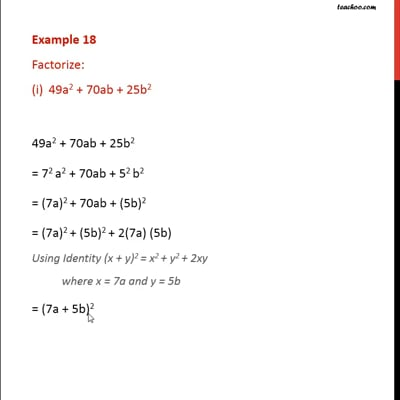Examples

Chapter 2 Class 9 Polynomials
Serial order wiseThis video is only available for Teachoo black users

Maths Crash Course - Live lectures + all videos + Real time Doubt solving!

### Transcript

Example 18 Factorize: 49a2 + 70ab + 25b2 49a2 + 70ab + 25b2 = 72 a2 + 70ab + 52 b2 = (7a)2 + 70ab + (5b)2 = (7a)2 + (5b)2 + 2(7a) (5b) Using Identity (x + y)2 = x2 + y2 + 2xy where x = 7a and y = 5b = (7a + 5b)2 = (7a + 5b) (7a + 5b)